## Example Questions

### Example Question #1 : How To Square A Decimal

Which quantity is greater? When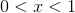Quantity AQuantity B

1

Quantity B is greater.

The two quantities are equal.

Quantity A is greater.

The relationship cannot be determined from the information given.

Quantity B is greater.

Explanation:

X must equal a number between 0 and 1.

If you square any decimal number in this range you will get an answer less than 1.  Try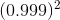= 0.998.

Therefore Quantity B is always larger.

### Example Question #2 : How To Square A Decimal

Which is greater, whenQuantity AQuantity B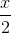Quantity A is greater

The relationship cannot be determined from the information given

Quantity B is greater

The two quantities are equal

The relationship cannot be determined from the information given

Explanation:

Whenmust be a decimal. Therefore it will decrease when it is squared. To find this answer we can substitute two values for.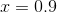and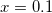When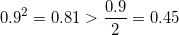But when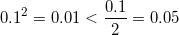Therefore we cannot tell which is greater based on the information given.

### Example Question #3 : How To Square A Decimal

Which is greater, whenQuantity AQuantity B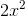Quantity B is greater

The relationship cannot be determined from the information given

The two quantities are equal

Quantity A is greater

The relationship cannot be determined from the information given

Explanation:

Whenmust be a decimal. Therefore it will decrease when it is squared. To find this answer we can substitute two values for.andWhen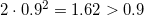But when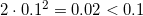Therefore we cannot tell which is greater based on the information given.

### Example Question #4 : How To Square A Decimal

Which is greater, when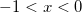Quantity A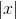Quantity BThe relationship cannot be determined from the information given

Quantity B is greater

The two quantities are equal

Quantity A is greater

Quantity A is greater

Explanation:

Whenmust be a negative decimal. Therefore it will decrease when it is squared and become positive. The first quantityis the distance to 0 from, in other words the positive value of. The second quantitywill always be positive, but because it is a decimal it will always be less than, ifwas a positive quantity. Since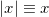, thenwiill always be greater than.

Quantity A is greater.

### Example Question #5 : How To Square A Decimal

Which is greater, whenQuantity AQuantity BQuantity A is greater

The two quantities are equal

The relationship cannot be determined from the information given

Quantity B is greater

Quantity B is greater

Explanation:

Whenmust be a negative decimal. Therefore it will decrease when it is squared and become positive. Becausemust be a decimal,will also be a decimal. Thereforewill never be greater than 1.

Quantity B is greater.

Tired of practice problems?

Try live online GRE prep today.